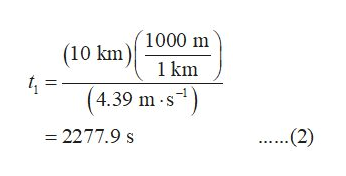# Hi, I am doing problem number 2 from the end of the chapter questions in my textbook. The problem reads as such:"An 18-year-old runner can complete a 10.0-km course with an average speed of 4.39 m/s. A 50-year-old runner can cover the same distance with an average speed of 4.27 m/s. How much later (in seconds) should the younger runner start in order to finish the course at the same time as the older runner?"I am looking at the solution for the problem and I did everything right except the last step. The solution says to subtract the time found for the 18 year old to run 10km from the time found for the 50 year old to run 10km. However, I divided the anwers I found. Why are we subtracting the answers from one another and not dividing them? Thank you!

Question

Hi, I am doing problem number 2 from the end of the chapter questions in my textbook. The problem reads as such:

"An 18-year-old runner can complete a 10.0-km course with an average speed of 4.39 m/s. A 50-year-old runner can cover the same distance with an average speed of 4.27 m/s. How much later (in seconds) should the younger runner start in order to finish the course at the same time as the older runner?"

I am looking at the solution for the problem and I did everything right except the last step. The solution says to subtract the time found for the 18 year old to run 10km from the time found for the 50 year old to run 10km. However, I divided the anwers I found. Why are we subtracting the answers from one another and not dividing them? Thank you!

check_circleExpert Solution
Step 1

Average speed is defined as the ratio of total distance covered to the total time taken. The expression for average speed is:

Step 2

Consider the time taken by the young runner to complete a distance d to be t1 and his average speed to be v1. Rearrange equation (1) in terms of time.

Step 3

Substitute 10 km for d and 4.39 m/s for v1 in...help_outlineImage Transcriptionclose(10 km 1000 m 4. (4.39 m s1) 1 km ...(2) - 2277.9 s fullscreen

### Want to see the full answer?

See Solution

#### Want to see this answer and more?

Solutions are written by subject experts who are available 24/7. Questions are typically answered within 1 hour*

See Solution
*Response times may vary by subject and question
Tagged in

### Physics